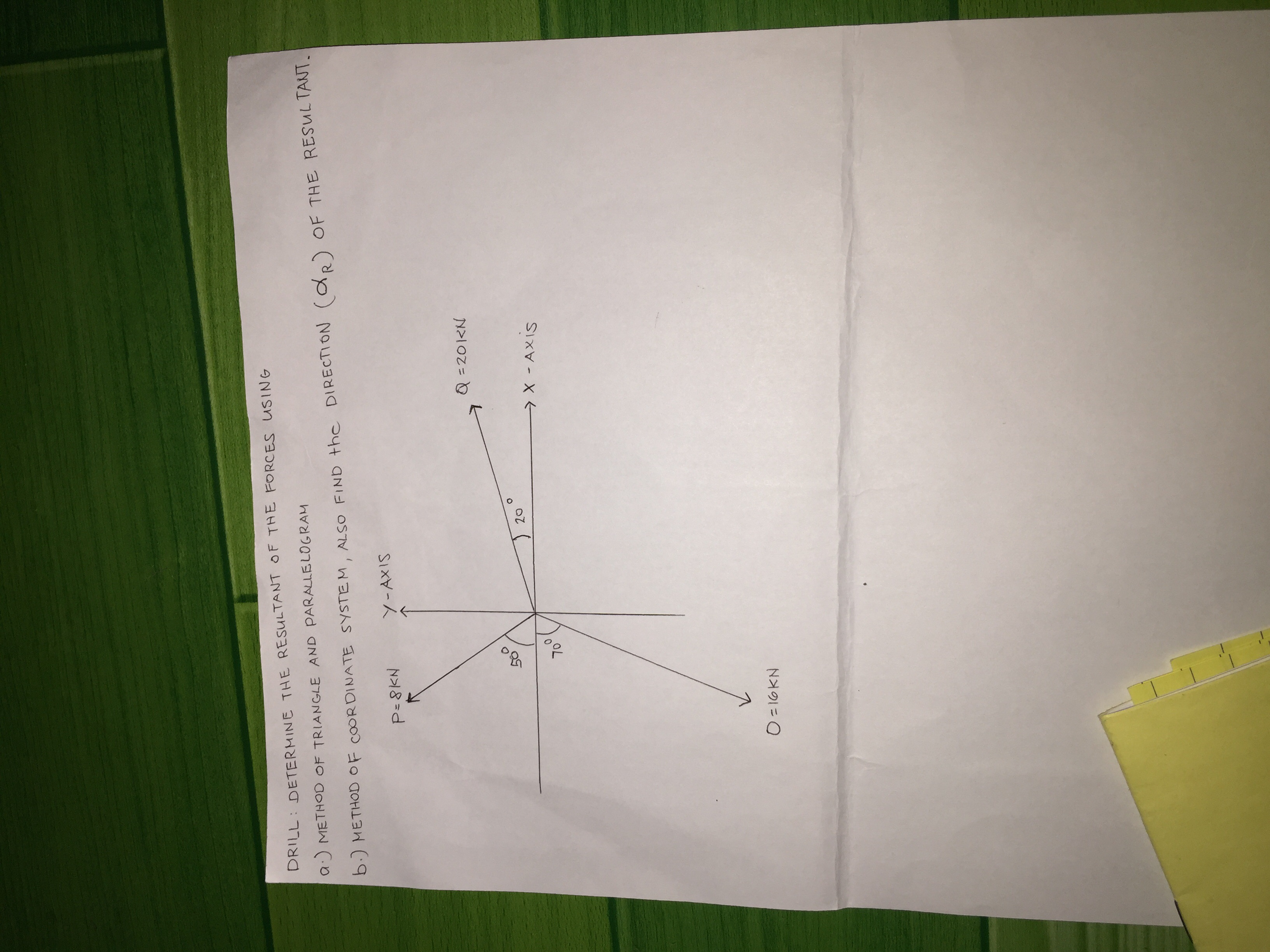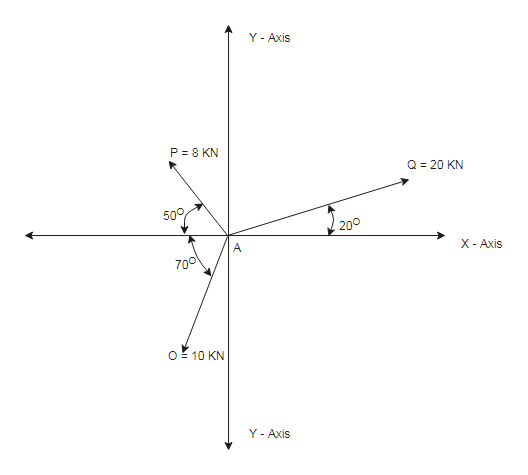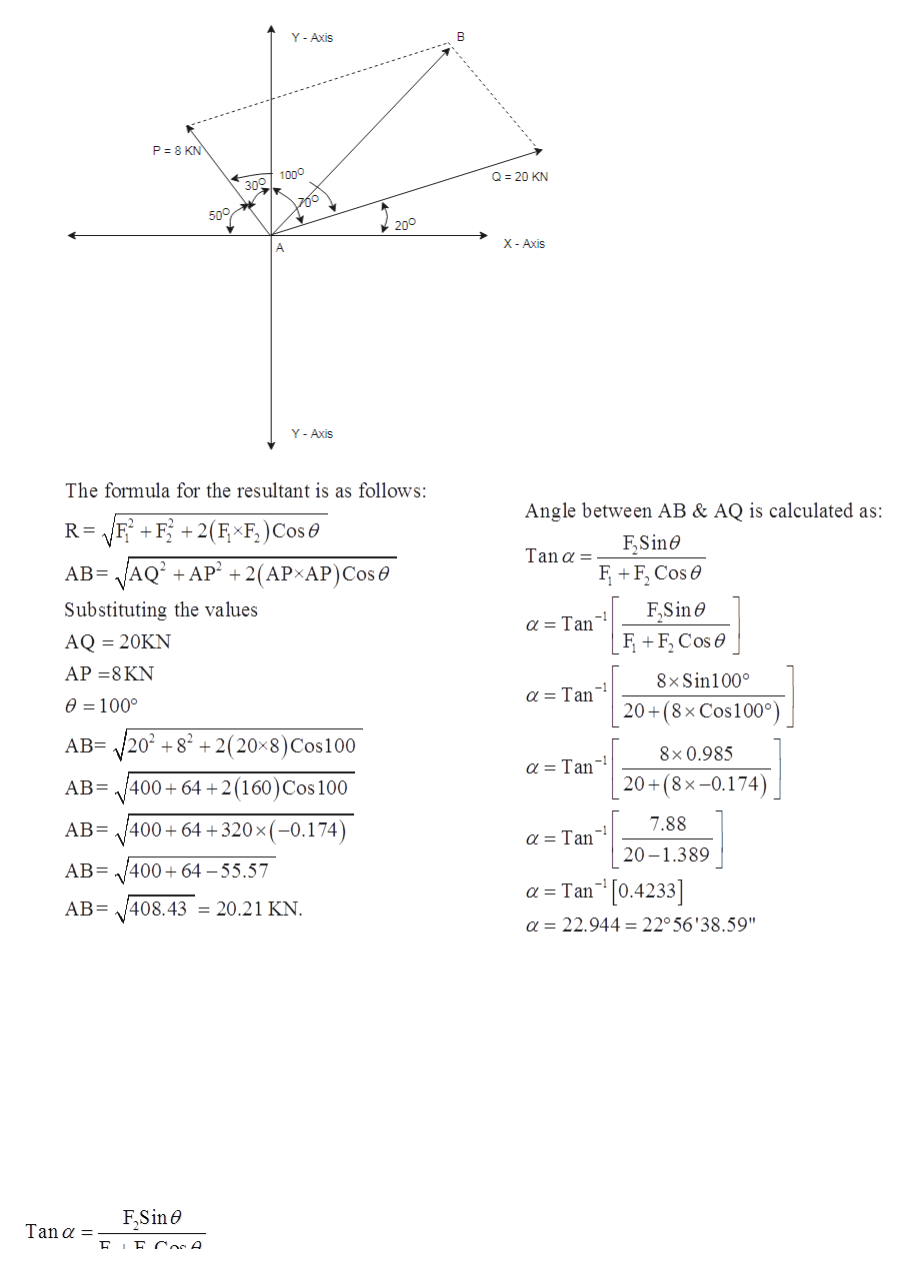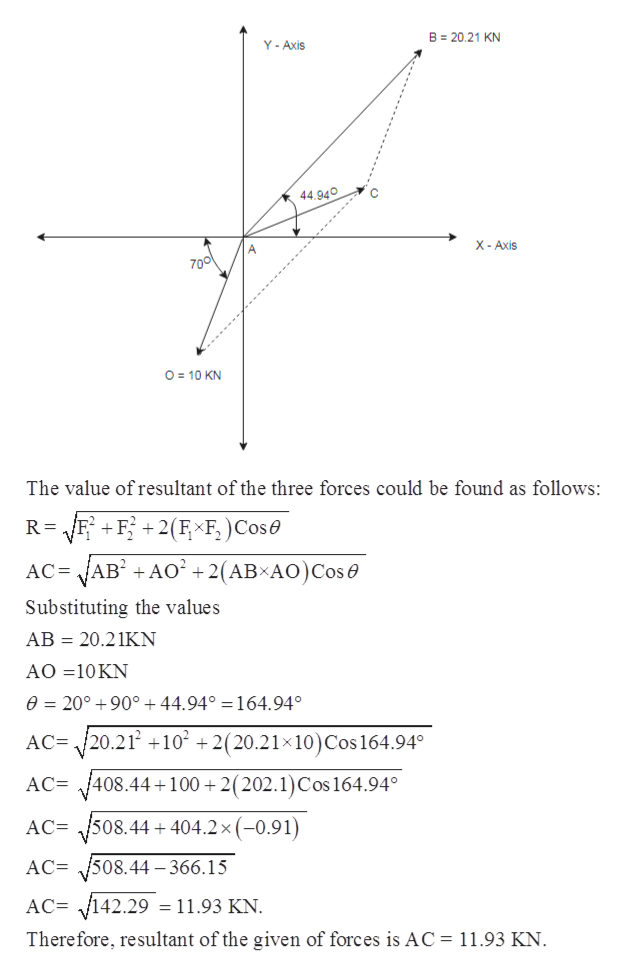# DRILL: DETERMINE THE RESULTANT OF TAE FORCES USINGa METHOD OF TRIANGLE AND PARALLELOGRAM(dR)OF THE RESULTANTb.) METHOD OF COORDINATE SYSTEM, ALSO FIND the DIRECTIONP-8KNY-AXIS=201KN20>X-AXIS70O=16KN

Question
12 viewshelp_outlineImage TranscriptioncloseDRILL: DETERMINE THE RESULTANT OF TAE FORCES USING a METHOD OF TRIANGLE AND PARALLELOGRAM (dR) OF THE RESULTANT b.) METHOD OF COORDINATE SYSTEM, ALSO FIND the DIRECTION P-8KN Y-AXIS =201KN 20 >X-AXIS 70 O=16KN fullscreen
check_circle

Step 1

To find

1. The resultant of given force system with parallelogram and triangle law of forces.
2. The magnitude and direction of resultant of the given force system using coordinate system.

We have been given the following information:help_outlineImage TranscriptioncloseY- Axis P 8 KN Q 20 KN 500 200 X - Axis A 700 O 10 KN Y- Axis fullscreen
Step 2

For part A.

The resultant of the given force system using the parallelogram law of forces.

Let’s first take forces AQ and AP.

Complete the parallelogram with diagonal representing the resultant of these two forces.

The following diagram would explain it betterhelp_outlineImage TranscriptioncloseY- Axis P 8 KN Q 20 KN 3n0 1000 500 200 X-Axis A Y- Axis The formula for the resultant is as follows: Angle between AB & AQ is calculated as: VFF2FF)Cos FSine Tan α= FF Cos -2(AP AP)Cos e AB AQ AP2 F.Sine F F Cos Substituting the values α Taη AQ 20KN АР -8KN 8x Sin100° a = Tan e =100° 20+(8x Cos100°) AB= /202 82+2(20x8) Cos100 8x0.985 a= Tan 20 (8x-0.174) 400 64 2(160)Cos 100 AB 7.88 400+ 64 +320 x(-0.174) AB= a = Tan 20 1.389 AB 400 64 55.57 a Tan 0.4233 AB 408.43 = 20.21 KN. a 22.944 22° 56'38.59" F.Sin Tan α fullscreen
Step 3

Now taking the resultant between AB and AP by applying t...help_outlineImage TranscriptioncloseB 20.21 KN Y-Axis C 44.940 X- Axis A 700 O 10 KN The value of resultant of the three forces could be found as follows: R /FF2F F, )Cos + AB +AO22(AB AO)Cos AC= Substituting the values AB 20.21KN AO 10 KN e 20° 90° +44.94° = 164.94 AC= 20.21 +10 2(20.21x10) Cos 164.94 AC 408.44+100 +2( 202.1) Cos 164.94° AC 508.44404.2 x (-0.91) 508.44-366.15 AC= AC= V142.29 = 11.93 KN Therefore, resultant of the given of forces is AC = 11.93 KN. fullscreen

### Want to see the full answer?

See Solution

#### Want to see this answer and more?

Solutions are written by subject experts who are available 24/7. Questions are typically answered within 1 hour.*

See Solution
*Response times may vary by subject and question.
Tagged in

### Civil Engineering